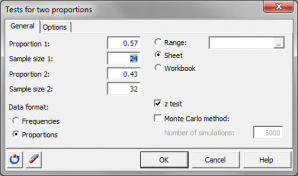# Test for two proportions

Tests for two proportions allow to test the difference between two observed proportions. Run them in Excel using the XLSTAT add-on statistical software.## The z-test to compare two proportions.

XLSTAT uses the z-test to compare two empirical proportions.

Let n1 be the number of observations verifying a certain property for sample S1 of size N1, and n2 the number of observations verifying the same property for sample S2 of size N2. The proportion of sample S1 verifying the property is defined by p1 = n1 / N1, and the proportion for S2 is defined by p2 = n2 / N2. Let D be the assumed difference (exact, minimum or maximum) between the two proportions p1 and p2. D is usually 0.

The test can be two-tailed:

• H0 : p1 - p2  = D
• Ha : p1 - p2 ≠ D

For the left-sided one-tailed test we have:

• H0 : p1 - p2  = D
• Ha : p1 - p2 < D

And for the right-sided one-tailed test we have:

• H0 : p1 - p2  = D
• Ha : p1 - p2 > D

This test is based on the following assumptions:

• the observations are mutually independent,
• the probability p1 of having the property in question is identical for all observations in sample S1,
• the probability p2 of having the property in question is identical for all observations in sample S2,
• the number of observations N1 and N2 are large enough, and the proportions are neither too close to 0 nor to 1.### analyze your data with xlstat

14-day free trial

Included in

Related features